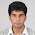### Drawing in the Air

Drawing in the air

For someone who is completely unaware about image processing, this project would seem something out of the world. The people with some understanding about how to read an image pixel by pixel would have some understanding about what I am doing here. I am building a program in JavaScript that takes input from the computer's webcam and create a tool that lets you draw in the air and that gets displayed on the computer. For this, we will have a special pen to which we code our program to detect.

To get started, let's use the <video> tag to receive the video feed from the camera, which I will later make invisible.. We'll also have two <canvas> tags that would each hold the current video frame and the drawing output from the tool respectively. We shall also have a button that clears data from the second canvas that has the drawing output.

<video autoplay="true" id="videoElement"></video>
<canvas id="canvas"></canvas>
<canvas id="drawingCanvas"></canvas>
<button onclick="clearFrameMemory()">Clear Drawing</button>

Let us assume that the video frame is of the size 500x420. The array named 'frameMemory' would store the data that shall be rendered on the 'drawingCanvas'. Let's define the function that would clear this array.

const width = 500;
const height = 420;
var frameMemory = [];
function clearFrameMemory(){
for(let i = 0; i < width*height*4; i++) {
if(i%4 === 3){
frameMemory[i] = 255;
} else {
frameMemory[i] = 0;
}
}
}
clearFrameMemory();

We make the video element start receiving data from the webcam using the following JavaScript code. Note that I have also made the video element display nothing as I am planning to make the first canvas display the same.

var video = document.querySelector("#videoElement");
video.style.display = "none";

.then(function (stream) {
video.srcObject = stream;
})
.catch(function (error) {
console.log("Something went wrong!");
});
}

The following bunch of code sets up the two canvases with the previously mentioned dimensions. It also stores the context of the canvases in different variables.

var canvas = document.querySelector('#canvas');
canvas.width = width;
canvas.height = height;
var context = canvas.getContext('2d');

var drawingCanvas = document.querySelector('#drawingCanvas');
drawingCanvas.width = width;
drawingCanvas.height = height;
var drawingContext = drawingCanvas.getContext('2d');

Now, we need to get the contents from the video at every frame. To do that, we attach an event listener on 'play' to the video element. The function named 'timerCallback' gets invoked from the event listener, which get's executed at every instance in a recursive, yet asynchronous manner, through 'setTimeout'.

var timerCallback = function timerCallback() {
if (this.video.paused || this.video.ended) {
return;
}
computeFrame();
setTimeout(function() {
timerCallback();
}, 0);
}

// video 'play' event listener
timerCallback();
}, false);

As you can see, the function 'timerCallback' invokes 'computeFrame' function at every instance. This is the function in which the main logic for the drawing tool goes in. Let me break that down. The first portion of the code simply sets the current video frame to the first <canvas>. Following that it iterates over all the pixels in the frame and checks for certain colors falling within the range - red < 20, green < 40 and blue > 45. In the curent lighting condition, I manually observed that this is the color of the pen that I am trying to use. Whereven this color is detected, we mark the 'frameMemory' and that is equivalently being displayed in the second <canvas>. We never clear the frame memory unless the clear function is invoked.

var computeFrame = function computeFrame() {
context.drawImage(video, 0, 0, canvas.width, canvas.height);
let frame = context.getImageData(0, 0, canvas.width, canvas.height);

// Lateral inversion (Mirror Image)
for (i=0; i<frame.height; i++) {
// We only need to do half of every row since we're flipping the halves
for (j=0; j<frame.width/2; j++) {
var index=(i*4)*frame.width+(j*4);
var mirrorIndex=((i+1)*4)*frame.width-((j+1)*4);
for (p=0; p<4; p++){
var temp=frame.data[index+p];
frame.data[index+p]=frame.data[mirrorIndex+p];
frame.data[mirrorIndex+p]=temp;
}
}
}
context.putImageData(frame, 0, 0, 0, 0, frame.width, frame.height);

// Getting the pixels for the pen
let l = frame.data.length / 4;
for (let i = 0; i < l; i++) {
let r = frame.data[i * 4 + 0];
let g = frame.data[i * 4 + 1];
let b = frame.data[i * 4 + 2];

if (r < 20 && g < 40 && b > 45) {
frame.data[i * 4 + 0] = 255;
frame.data[i * 4 + 1] = 255;
frame.data[i * 4 + 2] = 255;
frameMemory[i * 4 + 0] = 255;
frameMemory[i * 4 + 1] = 255;
frameMemory[i * 4 + 2] = 255;
} else {
frame.data[i * 4 + 0] = frameMemory[i * 4 + 0];
frame.data[i * 4 + 1] = frameMemory[i * 4 + 1];
frame.data[i * 4 + 2] = frameMemory[i * 4 + 2];
}
}

drawingContext.putImageData(frame, 0, 0);

return;
}

You can see how well this performs. To improve the performance, certain image processing techniques to filter the noise could be applied.

I hope you enjoyed this. I was originally intending on creating a three dimensional version of this, but due to COVID-19, I am unable to procure another webcam to perform this task. Maybe I will revisit this in the future.

1.This is lit

1.